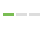# Scikit Learn 股票投资：p5## ▌内容

``````#获取文件全路径
full_file_path = each_dir+'/'+file
#读取html文件内容
source = open(full_file_path, 'r').read()
#将文件内容以':</td><td class="yfnc_tabledata1">'开始进行分割，表示分割后获取后面的第一个内容D/E的值
#然后再进行尾部分割，以'</td>'为中止
value = source.split(gather + ':</td><td class="yfnc_tabledata1">').split('</td>')
``````

## ▌源代码

``````import pandas as pd
import os
import time
from datetime import datetime

#获取数据的具体路径
path = '../intraQuarter'

#定义一个function，默认值为Total Debt/Equity (mrq)，以后可以更改为其他
def Key_Stats(gather="Total Debt/Equity (mrq)"):
statspath = path+'/_KeyStats'

#os模块提供的walk方法很强大，能够把给定的目录下的所有目录和文件遍历出来。
#方法：os.walk(path),遍历path，返回一个对象，他的每个部分都是一个三元组,('目录x'，[目录x下的目录list]，目录x下面的文件)
stock_list = [x for x in os.walk(statspath)]
#stock_list[1:] -- 主要作用是跳过根目录intraQuarter
for each_dir in stock_list[1:]:
#os.listdir(each_dir)：列出each_dir下的目录和文件
each_file = os.listdir(each_dir)
ticker = each_dir.split("\\")
if len(each_file) > 0:
for file in each_file:
#将文件名转换为时间序列
date_stamp = datetime.strptime(file, '%Y%m%d%H%M%S.html')
#转换为unix_time
unix_time = time.mktime(date_stamp.timetuple())

#获取文件全路径
full_file_path = each_dir+'/'+file
#读取html文件内容
source = open(full_file_path, 'r').read()
#将文件内容以':</td><td class="yfnc_tabledata1">'开始进行分割，表示分割后获取后面的第一个内容D/E的值
#然后再进行尾部分割，以'</td>'为中止
value = source.split(gather + ':</td><td class="yfnc_tabledata1">').split('</td>')
print(ticker + ":", value)
time.sleep(15)

Key_Stats()
``````